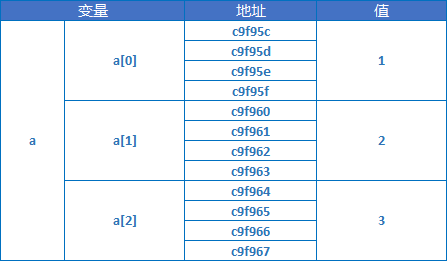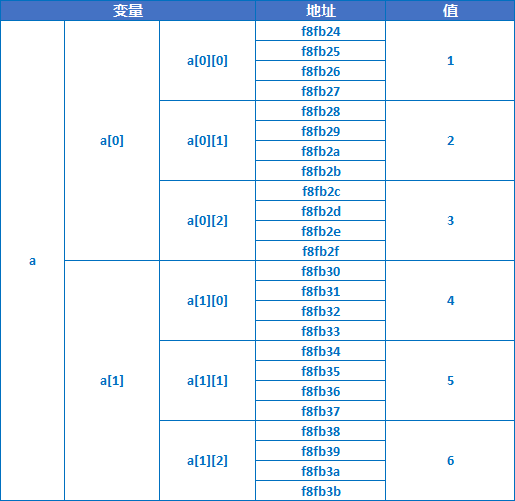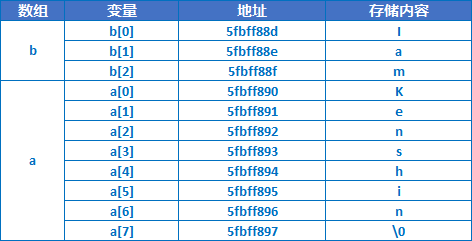# IOS开发之路--C语言数组和字符串

```#include <stdio.h>

int main(){
int len = 2;
//int a[len] = { 1, 2};//错误,不能使变量
int a;//正确
a = 1;
a = 2;
//a = 3;//超过数组长度，但是编译器并不会检查，运行报错
int b['a'] = {1,2,3};//'a'=97，所以可以作为数组长度，但是后面的元素没有初始化，其值默认为0
for (int i = 0; i < 97; ++i){
printf("b[%d]=%d\n",i,b[i]);
}
int c[2 * 3];//2*3是固定值可以作为数组长度
int d[] = { 1, 2, 3 };//如果初始化的同时赋值则数组长度可以省略，当前个数为3
}```

```#include <stdio.h>

int main(){
int const l = 3;
int a[l] = { 1, 2,3 };
for (int i = 0; i < l; ++i){
//由于当前在32位编译器下，int型长度为4个字节，可以判断出三个地址两两相差都是4
}
/*当前输出结果：
a = 1, address = c9f95c
a = 2, address = c9f960
a = 3, address = c9f964*/
}``````#include <stdio.h>

void changeValue(int a[]){
a = 10;
}

int main(){
int a = {1,2};
changeValue(a);
for (int i = 0; i < 2; ++i){
printf("a[%d]=%d\n",i,a[i]);
}
/*打印结果
a=10
a=2
*/
}```

```#include <stdio.h>

int main(){
int a;//2行3列，二维数组可以看成是一个特殊的一维数组，只是它的每一个元素又是一个一维数组
a = 1;
a = 2;
a = 3;
a = 4;
a = 5;
a = 6;
for (int i = 0; i < 2; ++i){
for (int j = 0; j < 3; ++j){
}
}
/*打印结果
*/
//初始化并直接赋值
int b = { { 1, 2, 3 }, { 4, 5, 6 } };
//由于数组的赋值顺序是先从第一行第一列，再第一行第二列...然后第二行第一列...，所以我们也可以写成如下形式
int c = { 1, 2, 3, 4, 5, 6 };
//也可以只初始化部分数据，其余元素默认为0
int d = { 1, 2, 3, 4 };
for (int i = 0; i < 2; ++i){
for (int j = 0; j < 3; ++j){
printf("d[%d][%d]=%d\n", i, j, d[i][j]);
}
}
/*打印结果
d=1
d=2
d=3
d=4
d=0
d=0
*/
//当然下面赋值也可以
int e = { {}, { 4, 5, 6 } };
//可以省略行号,但是绝对不可以省略列号，因为按照上面说的赋值顺序，它无法判断有多少行
int f[] = { {1,2,3},{4,5,6} };
}```a=a=&a a=&a

```//
// main.c
// ArrayAndString
//
// Created by KenshinCui on 14-7-06.
//

#include <stdio.h>

int main(int argc, const char * argv[])
{

char a[] = {'K','e','n','s','h','i','n','\0'};
printf("%s",a); //结果：Kenshin，注意使用%s输出字符串内容，如果换成整形输出格式其实输出的是a的地址
printf("\n");
printf("\n");
//后面的\0绝对不能省略,如果没有\0则会出现如下情况
char b[] = { 'I', 'a', 'm'};
printf("%s",b); //没有按照期望输出，多了一些垃圾数据，在当前环境打印结果：IamKenshin
printf("\n");
printf("\n");
//直接赋值为字符串，此时不需要手动添加\0，编译器会自动添加
char c[] = "Kenshin";
printf("c=%s",c); //结果：c=Kenshin
printf("\n");

//二维数组存储多个字符串
char d={"Kenshin","Kaoru","Rose","Jack","Tom","Jerry"};

return 0;
}
``````//
// main.c
// ArrayAndString
//
// Created by Kenshin Cui on 14-7-04.
//

#include <stdio.h>

int main(int argc, const char * argv[])
{
/*字符操作*/
putchar('a'); //结果：a，putchar一次只能输出一个字符
printf("\n");
putchar(97);//结果:a
printf("\n");
char a;
a=getchar();//getchar()一次只能接收一个字符，可以接收空格、tab、回车
printf("a=%c",a);
printf("\n");

/*字符串操作*/
char b[]="Kenshin";
printf("b=%s",b);
printf("\n");
puts(b); //puts用于输出单个字符串，不能像printf格式化输出，会自动添加换行
printf("\n");

char c;
scanf("%s",c);//注意c没必要写成&c，因为c本身就代表了数组的地址
printf("c=%s\n",c);//注意即使你输入的内容大于10，也能正确输出，但是下面的gets()函数却不行
printf("\n");

//gets()函数，注意它是不安全的，因为接收的时候不知道它的大小容易造成溢出，建议不要使用
char d;
gets(d); //gets一次只能接收一个字符串，但是scanf可接收多个；scanf不能接收空格、tab，gets则可以
printf("d=%s",d);
printf("\n");

char e[]={'K','s','\0'};
printf("%lu",strlen(e)); //结果是：2，不是3，因为\0不计入长度
printf("\n");
char f[]={"Kenshin"};
printf("%lu",strlen(f)); //结果是：7
printf("\n");

char g;
strcpy(g,"hello,world!");
printf("%s",g); //结果是：hello,即使定义的g长度为5，但是也能完全拷贝进去
printf("\n");
char h;
char i[]={'a','b','c','\0','d','e','f','\0'};
strcpy(h,i);
printf("%s",h); //结果是：abc,遇到第一个\0则结束
printf("\n");

strcat(i,"ghi");
printf("%s",i); //结果是：abcghi,注意不是abcdefghi,strcat，从i第一\0开始使用“ghi”覆盖，覆盖完之后加上一个\0,在内存中目前应该是：{'a','b','c','g','h','i','\0','f','\0'}
printf("\n");

char j[]="abc";
char k[]="aBc";
char l[]="acb";
char m[]={'a','\0'};
printf("%d,%d,%d",strcmp(j,k),strcmp(k,l),strcmp(l,m));//遇到第一个不相同的字符或\0则返回两者前后之差，结果：32,-33,99
printf("\n");

return 0;
}```

#### 您可能感兴趣的文章: